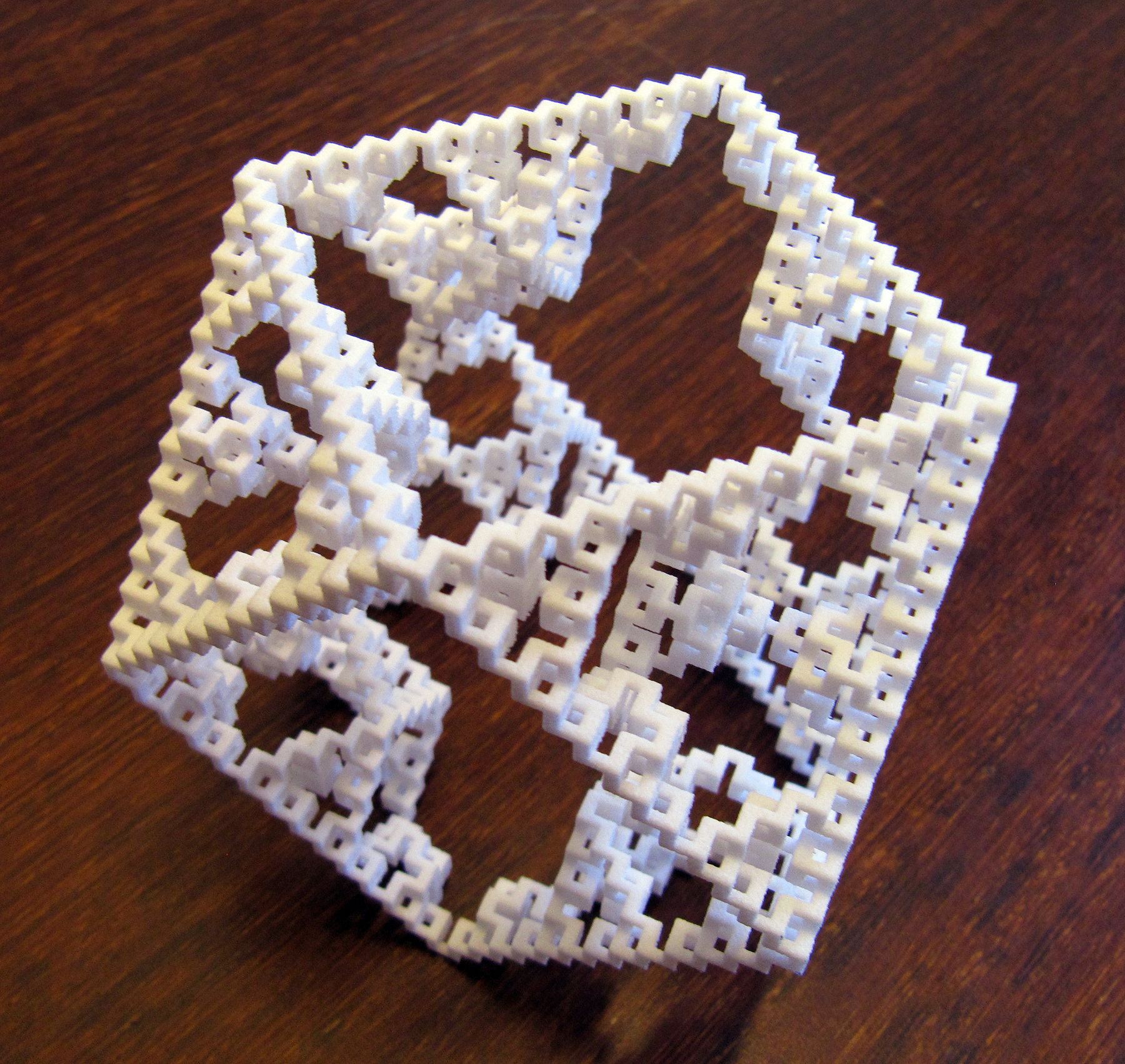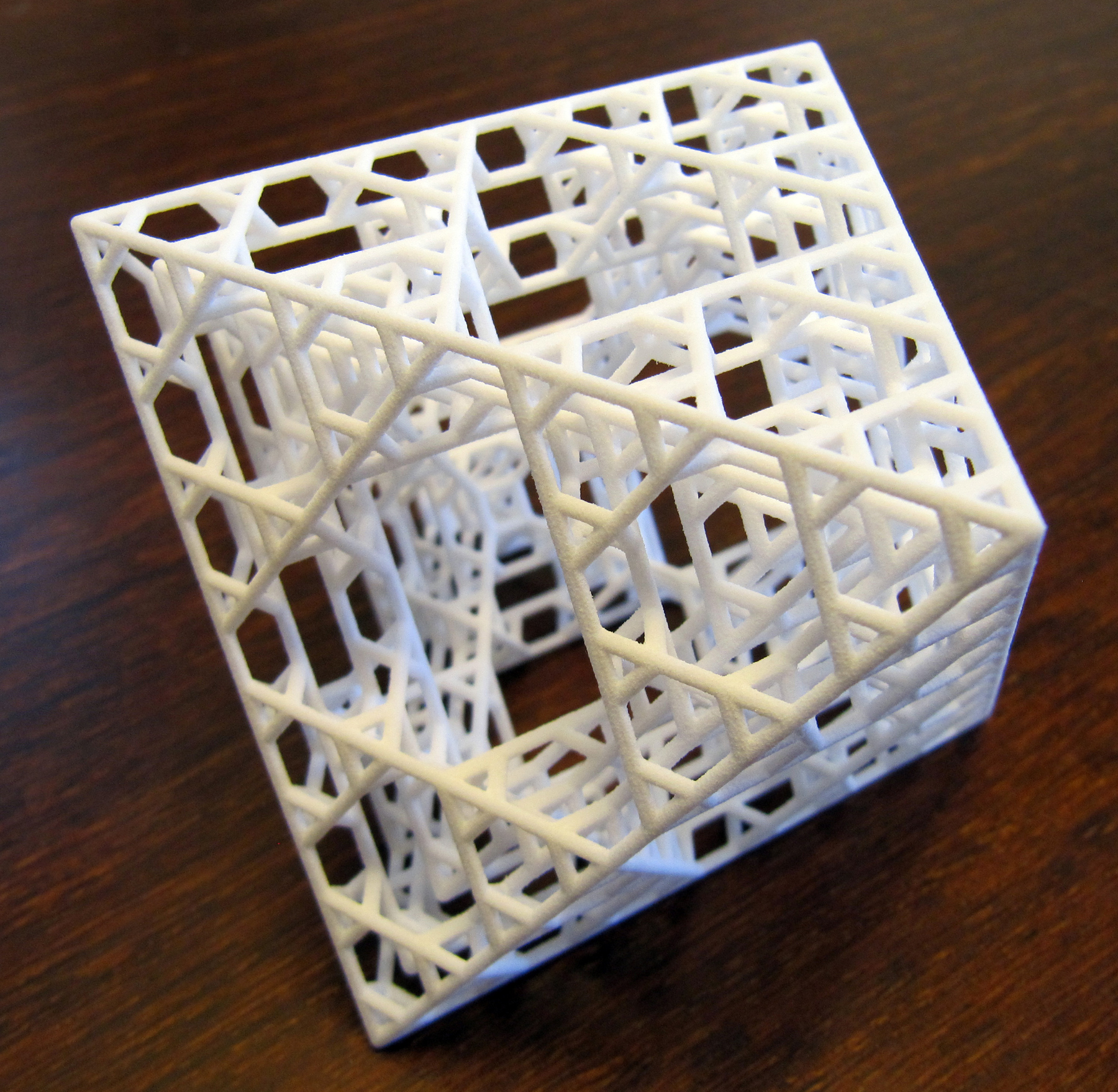# Henry Segerman

Research Fellow
Department of Mathematics and Statistics, University of Melbourne
Melbourne, Australia

Henry Segerman is a postdoctoral mathematician. His mathematical research is in 3 dimensional geometry and topology, and concepts from those areas often appear in his work. Other artistic interests involve procedural generation, self reference, ambigrams and puzzles.Cuboctahedral fractal graph
66 x 66 x 66 mm
PA 2200 Plastic, Selective-Laser-Sintered
2010

This is a graph embedded in 3-dimensional space as a subset of the cubic lattice. The graph has a fractal structure, formed by a process of repeated substitution. Each vertex at each step of the construction is degree 3, and is replaced at the next step by 7 vertices which can be thought of as a subset of a 3 x 3 x 3 cube, with certain choices of edges connecting them to each other. Each edge is replaced at the next step by a single edge, joining to the vertex in the centre of each 3 x 3 face. We begin the construction with the first step being the edges of a cube, and this is the result at the fourth step.Octahedron fractal graph
103 x 103 x 103 mm
PA 2200 Plastic, Selective-Laser-Sintered
2010

This is a graph embedded in 3-dimensional space as a subset of an "octahedral lattice", which is related to the tessellation of space using octahedra and tetrahedra. The graph has a fractal structure, formed by a process of repeated substitution. Each vertex at each step of the construction is degree 4, and is replaced at the next step by 6 vertices arranged in an octahedron, with certain choices of edges connecting them to each other. Each edge is replaced at the next step by 2 parallel edges. We begin the construction with the first step being the edges of an octahedron, and this is the result at the fourth step.Space filling graph 1
68 x 68 x 68 mm
PA 2200 Plastic, Selective-Laser-Sintered
2010

This is a graph embedded in 3-dimensional space as a subset of the cubic lattice. The graph has a fractal structure, analogous to the fractal structure of a step in the construction of a space filling curve, but with greater connectivity. This greater connectivity makes the physical sculpture considerably more robust than the analogous sculpture of a step in the construction of a space filling curve would be. Each vertex at each step of the construction is degree 3, and is replaced at the next step by 8 vertices arranged in a 2 x 2 x 2 cube, with certain choices of edges connecting them to each other. Each edge is replaced at the next step by 4 parallel edges. We begin the construction with the first step being the edges of a cube, and this is the result at the fourth step. The spacing between the vertices varies in order to highlight the fractal structure.